# Column choice and optimization

Abstract Column choice is governed by three important requirements: separation power, sample capacity and speed of the column.
These parameters are not independent. This means that if we want a column with maximum separation power, the column will not be 'fast' and/or does not have a large sample capacity. A fast column, on the other hand, has less separation power and/or a small sample capacity. This articles explains the details and provides tips and rules of thumb.

LevelBasic

Column choice is governed by three important requirements:

Optimization targetsIf more requirements for a particular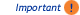application are made, this will lead to a compromise.

 Column choice criteria: Type of stationary phase: Polarity Amount of stationary phase Film thickness Column dimensions Length (efficiency) Internal diameter GC system parameters: Column flow Type of carrier gas Oven temperature, temperature programming

Column choice and optimization requires a systematic approach with respect to these criteria.

## The choice of stationary phase

The separation of two components (the resolution) is the result of three conditions.

1. Components must be retained on a stationary phase (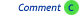retention)
2. Components must be retained differently (selectivity)
3. The peaks must be sufficiently narrow in order to avoid/reduce overlapping (efficiency)

The polarity of the stationary phase is of major importance. This fact can be translated into the basic rule for the choice of stationary phase: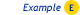'Like dissolves Like'. This rule must be interpreted as follows:

• The best (larger) retentions are obtained if the sample components correspond chemically to the stationary phase.
• The best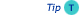selectivity is obtained if optimum use is made of the differences in chemical/physical properties of various components.

 Stationary phase optimization 'Boiling point'-separation on non-polar phase 'Polarity'-separation on polar phases General rule: "LIKES LIKE" Sample Stationary phase Alcohols Polyglycols (CP-wax 52 CB) Hydrocarbons Methyl silicone (CP-Sil 5 CB) Esters Polyesters (DEGS) Fatty acids FFAP

The function of the stationary phase in GC is to selectively and sufficiently retain sample components in a mixture in order to produce the required separation.

Important separation parameters are:

Theselectivity factor has the most critical effect of these three parameters, affecting resolution according to the resolution equation.

It is not surprising that the correct choice of stationary phase can be a very important factor for difficultseparations.

## Stationary phase polarity

The availability of a great variety of stationary phases is not necessary for the majority of applications and it is usually sufficient to choose a stationary phase with similarpolarity characteristics to those of the sample components. Strong dissimilarity between stationary phase and sample component polarities will result in earlierelution of the sample components than expected.

Despite many attempts to develop systems for stationary phase selection, the choice of the optimum phase is still largelytrial and error. Well-known attempts to categorise phases are theRohrschneider system, theMcReynolds system and the Kovats retention index. In practice their applicability is limited. TheKovats index provides little information about the respective polarities of the solute and stationary phase, but by comparing values measured on two stationary phases a direct quantitative measurement of the relative polarity can be obtained.

Increasing the polarity of the stationary phase will increase the selectivity of the column for polar components in the sample. However, the actual selectivity of a stationary phase for a given mixture of compounds cannot be predicted from its polarity and has to be measured experimentally.

The figures below show the (strong) influence of stationary phase polarity on the selectivity for a specific test sample. As an example, the peak pair 8 and 9 is just baseline separated on the first column. On the second column, the resolution is much better, whereas on the third column the distance between the peaks 8 and 9 is very large. Which column is the best will also clearly depend on the other compounds present in the sample.

Column choice (selectivity)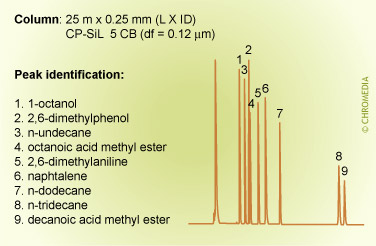Column choice (selectivity)Column choice (selectivity)Phil’s Tips: When looking at these 3 chromatograms above, compare the results for relative retention by observing how peaks shift relative to each other for the different conditions. For example, check positions of peaks 8 and 9. Alkane 8 is much less retained on the more polar phase (CP Sil 19 CB), because it is less compatible with this phase. We can interpret this as being due to lower stationary phase solubility (distribution coefficient) which reduces solute concentration in the carrier phase and elutes it earlier.

## Film thickness, temperature and retention

In addition to the stationary phase characteristics and the nature of the sample components, the retention factor k is determined by 3 other parameters:
• Amount of stationary phase (film thickness of the column)
• Column diameter
• Column temperature

The first two parameters are combined in the expression for the phase ratio β in terms of column volume (gas phase) and stationary phase volume (film thickness).

The relation between the retention factor k and the phase ratio is described by:

k = K /  β

where K describes the physical equilibrium of the component between the gas- and the stationary phase in the column. This factor K is thetemperature-dependent component in the complete separation process, described in the van ‘t Hoff equation.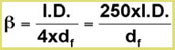Film thickness and retention (constant temperature)Chromatographic selectivity depends on the characteristics of both the stationary phase and of the solute molecules. Similar compounds, consisting of molecules of the same chemical type and having very similar boiling points, are difficult to separate on a non-polar phase unless very high efficiency columns are used.

In a chromatogram of aromatic and aliphatic hydrocarbons on a low polarity column (CP-Sil 5 CB) the componentselute in strict order of boiling point and the separation is much as one would predict from Raoults law.

A similar effect is shown on a different column containing the same phase for a separation of a mixture of solvents having different polarities. The alcohols now elute from the column relatively faster than any of the other compounds. The elution order is: methanol (BP 65°C) and ethanol (BP 78°C) before acetone (BP 56°C) and isopropanol (BP 82°C) before methylene chloride (BP 40°C). The other solvents also elute mostly according to their polarities. Methyl isobutyl ketone (BP 119°C) elutes well before toluene (BP 110°C).

The elution order now depends on both the relative vapour pressures and the differences in polarity.

Elution order of solventsIn practice it is very difficult to predict the elution order of compounds on neutral, non-polar columns, only based on boiling points, if these compounds are different in polarity and physical properties.

Boiling point separation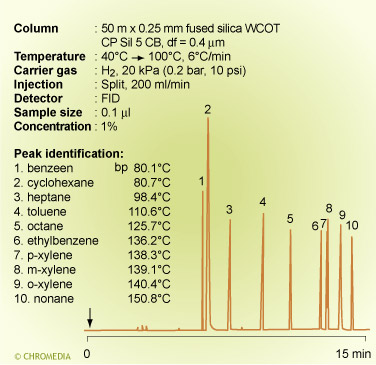On polar stationary phases the retention order can only be found empirically.

## Film thickness and temperature selection

The amount of stationary phase affects other separation parameters:

The amount of stationary phase has a twofold effect on the resolution

• Retention factor (k value) of the sample components: the more stationary phase, the larger the k value. Increasing the k values by means of an increase of the amount of stationary phase can contribute positively to the separation. Also the column diameter plays an important role, so in fact the phase ratio β is the dominant factor in the retention mechanism at constant temperature.

If the k values are large enough (> 5), the relative effect on the resolution is small. The contribution of the k- value in the resolution equation is then near to 1. More stationary phase (thicker film) will then have very little effect.

Effect retention on resolutionThese two effects oppose each other:

• A large amount of stationary phase results in large k-values which have a positive effect on resolution.
• A large amount of stationary phase, on the other hand, results in less efficiency (fewer plates).

The amount of stationary phase has a direct effect on the retention values. Retention can, in principle, also be increased by reducing the oven temperature.

The following method can be applied:

Athin film column is, in view of the efficiency, always preferred to a thick film. Try to select the oven temperature with a thin film in such a way that the sample components get a suitable k-value. This is dependent on the volatility of each component. In practice these will be between 0 and 5 for a simple sample and between 0 and 20 for a complex sample. In case (and that is only valid for very volatile samples) that despite the very low oven temperature, the k-values are too small, choose a thicker film.

The following rule of thumb can be formulated for the choice of a thin-film or thick-film column:

• For volatile components it would be best to choose a high loading (thick film). The profit in resolution due to the increase of the k-values counterbalances the loss in efficiency.
• The amount of stationary phase should be kept as small as possible with high boiling components. Especially columns with β < 100 should be avoided.

 Features thick-film columns Advantages: High k-values for volatile compounds High sample capacity Highly inert, even for polar compounds (acids, bases) Disadvantages: Lower efficiencies More bleeding Lower optimum gas velocity

Film thickness and retention (temperature programmed analysis)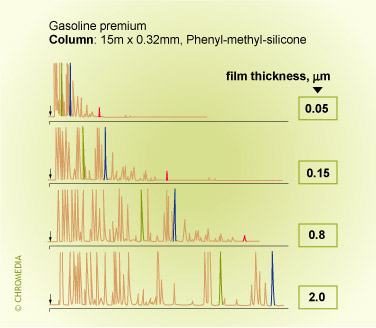## Column length

Open tubular columns normally operate at low pressure drops. The theoretical plate number is directly proportional to column length, assuming that other factors such as diameter and film thickness remain constant.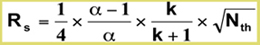Resolution depends on:

• α : selectivity factor
• k: retention factor
• Nth: (theoretical) plate number

The resolution between any two sample components is proportional to the square root of the plate number and therefore, increasing the column length only has a weak effect, which means that:

The result of replacing a 25m x 50m column is an ill-advised attempt to achieve baseline resolution between the two compounds shown. The compounds would still not be resolved to baseline, the separation time would be doubled, and the peak heights would be smaller.

Resolution as function of column length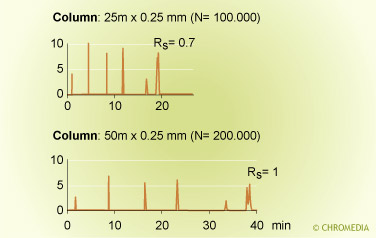## Efficiency of the column

The efficiency of a capillary column is determined by three column parameters:

1. Amount of stationary phase
The Cs-term and Cm-term in the Golay equation clearly show the effect of amount of stationary phase on separation efficiency.
2. Column ID
A thin-film column has a higher plate number than a thick-film column. A narrow-bore column provides smaller plate heights than a wide-bore column.
3. Column length
The plate number is proportional to the column length. Long columns provide high separation power.

Column diameter and efficiency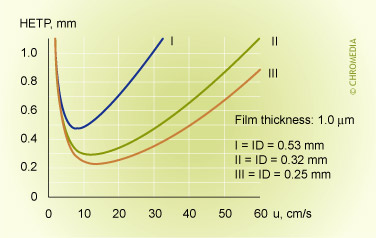Film thickness and efficiencyIn addition to these three column parameters, there are a number of GC system parameters which can affect the efficiency and analysis time:

• Type of carrier gas
The type of carrier gas affects mainly the analysis time of the separation on a given column. In the Golay equation for capillary columns, in which the plate height is represented as a function of the linear gas velocity, the diffusion coefficient Dm is present both in the B-term (i.e. the longitudinal diffusion term) and the Cm-term (i.e. the resistance to mass transport in the mobile phase).

As the Dm is a function of thetype of carrier gas (for 'light' carrier gases, such as helium and hydrogen, it is 3 to 4 times larger than for 'heavy' carrier gases, such as nitrogen and argon), it means that the shape of the H-u curve, and thus the efficiency, is dependent on the type of carrier gas.

• Linear gas velocity
The location of the optimum in the gas velocity, as well as the slopes before and after this optimum, are different for various gases. However, if the Cs-term is negligible (as with thin-film columns) then the diffusion coefficient Dm cancels out in the equation for the minimum plate height and therefore the choice of carrier gas has virtually no effect on the column efficiency at optimum velocity. But, as emphasised above, the velocity at which the optmum occurs is much more favourable for the lighter hydrogen and helium carrier gases.

van Deemter profile of helium and nitrogen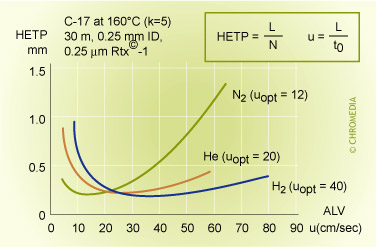From the different H-u curves it is evident that 'heavy' carrier gases in the optimum generate slightly higher efficiencies. However, this optimum is obtained at a low gas velocity and the analysis time is longer. Increasing the gas velocity for nitrogen, in view of the slope of the curve, will result in a considerable loss of plates. Using helium and hydrogen will result in much less loss of plates if the analysis has to be carried out faster than the optimum gas velocity. Helium or hydrogen are generally a better choice.

'H-u' curves of different carrier gasesHelium and hydrogen in particular, offer advantages of short analysis times. Hydrogen compared tonitrogen can reduce the analysis time by a factor of 3. It is evident that the relatively high efficiency of hydrogen at high velocities is only valid if there are no disturbing factors, such as safety rules.

Effect of carrier gas type on analysis time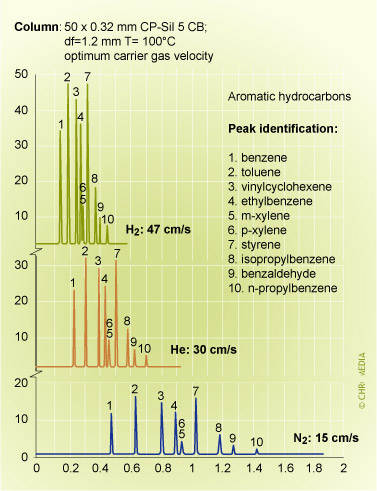Note: The use of H2 here gives about the same separation performance, but in much less time, due to higher optimum carrier flow rate.

## Temperature effects on retention and selectivity

The effect of temperature on the analyte partition coefficient is given by the van’t Hoff relationship: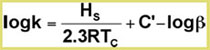Where Hs=molar heat of solution of solute;

R = Gas constant

Tc= Column temperature (degrees K)

C’ = Integration constants, and column phase ratio β

A plot of log (k) versus 1/Tc should be linear with a slope = Hs/2.3R, provided Hs and C' are constant.

Some experimental plots for a number of compounds on three phases of different polarities are shown below.

Retention factor vs temperatureRetention factor vs temperature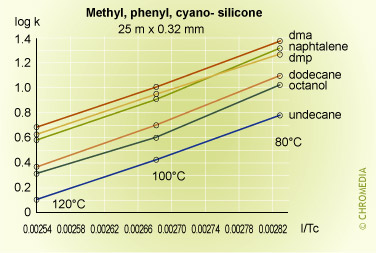The plots are linear over the range of temperatures employed, and lie approximately parallel to each other indicating that there is little change in selectivity with temperature for many compounds particularly if they are chemically similar to each other. In fact selectivity usually increases very slowly with decreasing temperature but to take advantage of this a considerable sacrifice would have to be made in analysis time, as shown in the theoretical plot of resolution versus column temperature shown for a boiling point difference of 1 degree. An exception to this rule was for the naphthalene van 't Hoff plot, which gave a smaller slope than the other components, and consequently intersected that for dimethylphenol. This means that the peaks for these two compounds will reverse their elution order at about 85oC on a stationary phase containing cyano-groups (CP-Sil 19 CB).

This kind of behavior is often experienced when compounds of different chemical types are separated on moderate to high polarity stationary phases, and cannot be predicted from runs at a single temperature. Difficult separations should always include a systematic study at various temperatures to determine optimum conditions for the proposed method.

As the temperature increases from 60°C, the resolution falls rapidly due to the effect of temperature on the selectivity factor, and at about 85°C the two components co-elute. Resolution then increases rapidly after the reversal in the elution order, reaching a maximum at about 160°C. After this the resolution starts to decline because of the reducing retention factor and its effect on the k/(1+k) function.

The logarithmic relationship between temperature and retention shows that accurate and reproducible control of column temperature is essential. An approximate guide to the magnitude of the effect of column temperature can be derived from the log (k) equation. For most compounds and column temperatures the result is similar, i.e. that a change in column temperature of 20°C will change k by a factor of about 2.

## The speed of capillary columns

An important feature of capillary GC columns is the speed, in other words, fast analysis. Analysis time is much shorter than with packed columns, combined with a better separation and peak shape.

Capillary columns are relatively long, but this does not necessarily result in long analysis times.

The optimum gas velocity can be calculated from the Golay equation:

Uopt =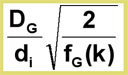Theoptimum gas velocity, and therefore the speed of analysis, is inversely proportional to the column diameter! Fast analysis can be achieved by using short columns with small internal diameters. This combination is often known as fast GC or high speed GC.